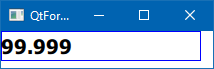# problem to send a double through a TextInput

• Hello everyone,

Like I said, I would like to retrieve a double from a TextInput but I retrieve only the rounded value.

I show you my code :

``````Rectangle{
color : "green"
height : parent.height/4
width : parent.width

TextInput{

id: idTextInput
inputMethodHints: Qt.ImhFormattedNumbersOnly
text: controller.value
font.pixelSize: 13
font.bold: false
color: "black"
anchors.verticalCenter: parent.verticalCenter
anchors.horizontalCenter: parent.horizontalCenter
validator: DoubleValidator{bottom: 0 ;
top: 100;
decimals : 3
notation: DoubleValidator.StandardNotation}

onAccepted: {
controller.newValuefromTextInput( parseFloat(text) )
controller.value = parseFloat(idTextInput.text)
console.log("value  = " + text)
}

}
}
``````

and the methode newValuefromTextInput from controller is :

``````void Controller::newValuefromTextInput(double v)
{
qDebug() << "value = " << v ;
}

Do you have an idea of the problem?

Thanks
``````

• Hi @cosmoff

Code looks fine, it do print the double value in the C++

• QML
``````        TextInput {
id: idTextInput

focus: true
anchors.fill: parent
verticalAlignment: TextInput.AlignVCenter
font {
pointSize: 15;
bold: true;
}

validator: DoubleValidator {
top: 100; // 100 is the maximum acceptable value (More than 100 its not acceptable & onAccepted will not be called).
bottom: 0;
decimals: 3;
notation: DoubleValidator.StandardNotation
}

onAccepted: {
console.log("value  = " + text)
cppObject.doubleValueQML(idTextInput.text)

}
}
``````
• main.cpp
``````    engine.rootContext()->setContextProperty("cppObject", &cppClass);
``````
• MyClass.h & MyClass.cpp - I am using `public slots` function to `QML`
``````public slots:
void doubleValueQML(double value);

void MyClass::doubleValueQML(double value)
{
qDebug() << Q_FUNC_INFO << value << endl;
}
``````

Output:

``````QML debugging is enabled. Only use this in a safe environment.
qml: value  = 100.000
void MyClass::doubleValueQML(double) 100

qml: value  = 99.99
void MyClass::doubleValueQML(double) 99.99

qml: value  = 99.100
void MyClass::doubleValueQML(double) 99.1

qml: value  = 99.001
void MyClass::doubleValueQML(double) 99.001

qml: value  = 99.999
void MyClass::doubleValueQML(double) 99.999
``````

All the best.

I do not understand how you code can work because you insert a string in your function MyClass::doubleValueQML(double) which need a double. So I put a parseFloat() But like I said the function seems to round the value

• parseFloat

I tried both the ways, It works properly even with `parseFloat(idTextInput.text)`

``````            onAccepted: {
console.log("value  = " + text)
cppObject.doubleValueQML(parseFloat(idTextInput.text))
}
``````
• Output:
``````QML debugging is enabled. Only use this in a safe environment.
qml: value  = 11.11
void MySpinBoxValue::doubleValueQML(double) 11.11

qml: value  = 22.22
void MySpinBoxValue::doubleValueQML(double) 22.22

qml: value  = 99.100
void MySpinBoxValue::doubleValueQML(double) 99.1

qml: value  = 99.999
void MySpinBoxValue::doubleValueQML(double) 99.999
``````Yes I understood my problem. In fact, because of validator I cannot fill the textInput with the character ' . ' for the float like 70.5 but only 70,5 and so Qt does not understand the character ' , '

Do you have an idea why my validator do not want the character ' . '

• Do you have an idea why my validator do not want the character ' . '

localization issues.

I know of 3 ways to solve this.

``````onAccepted: {
var modifiedText = text.replace(",",".")
controller.newValuefromTextInput( parseFloat(modifiedText) )
controller.value = parseFloat(modifiedText)
console.log("value  = " + text)
}
``````

• @J.Hilk

yes it works with : var modifiedText = text.replace(",",".")

thanks a lot